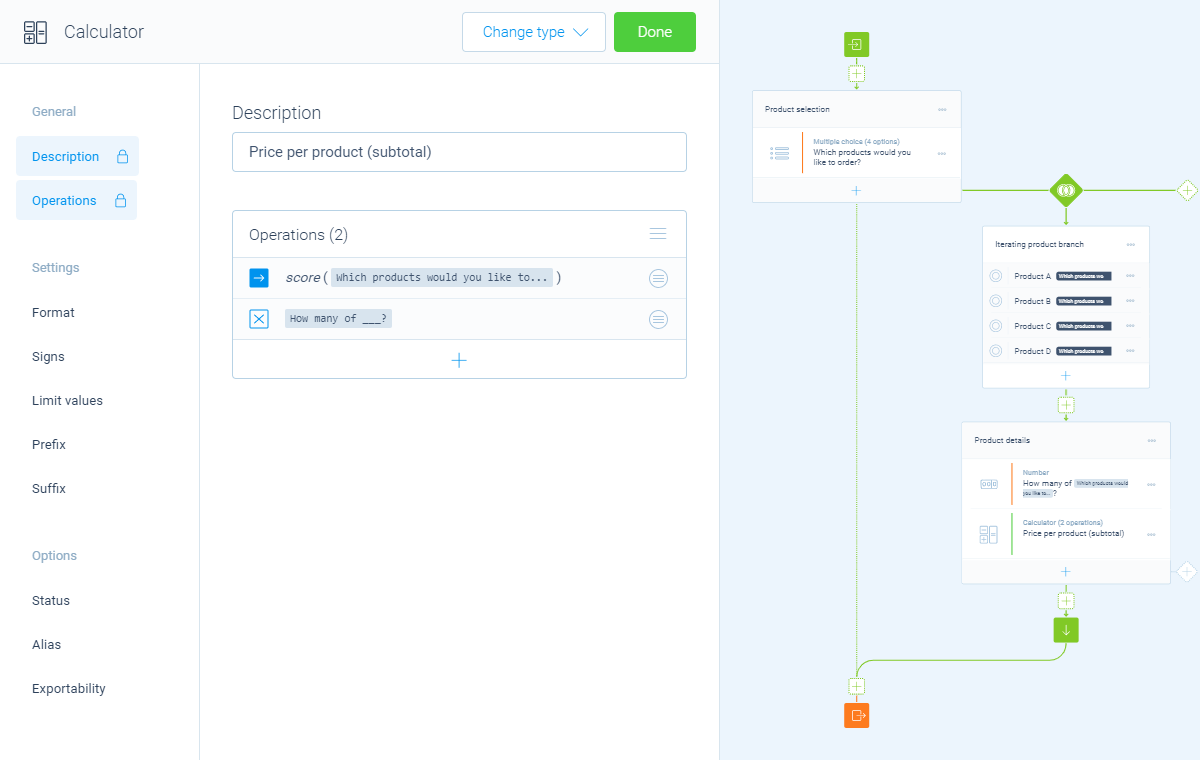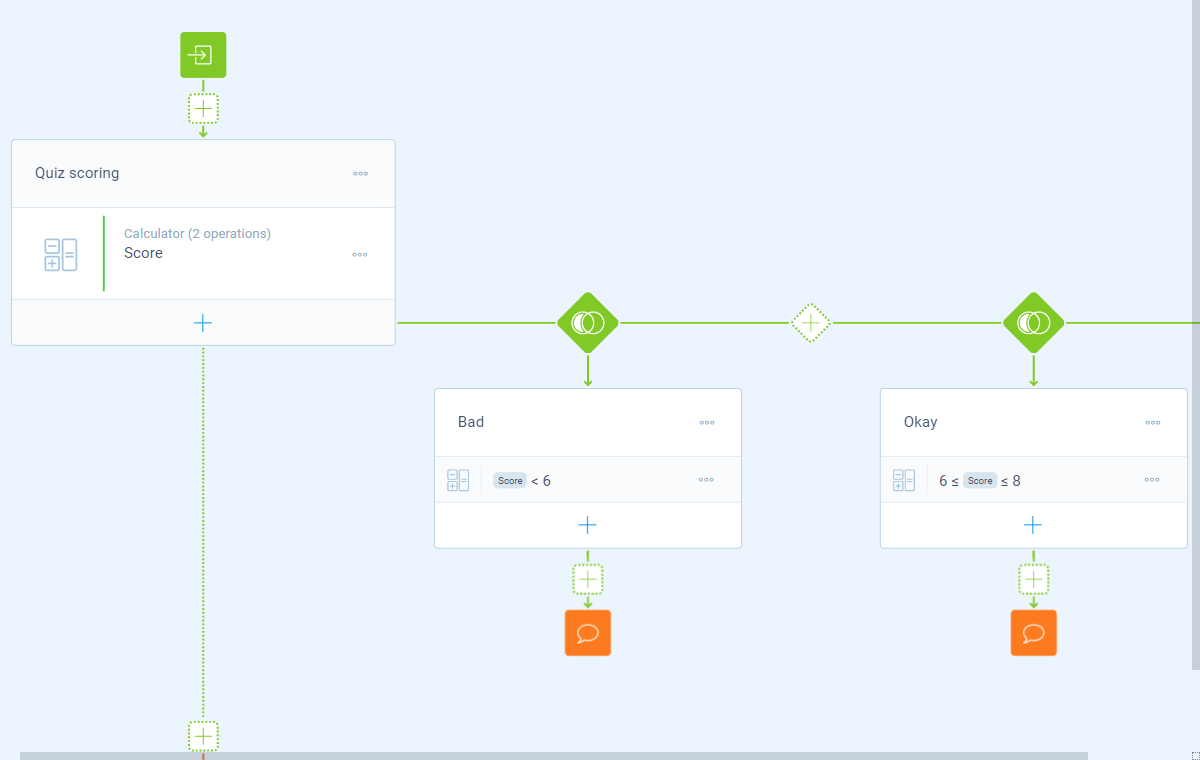Using logic3 Min.

# How to use calculations with logic branches

You can use all calculations in combination with any logic branch in your form. This makes your calculations even more smart and powerful!

## When to use

Logic is an important part of forms in Tripetto. Of course the calculator block blends perfectly with the logic branches in your form. This enables you to do all kinds of smart actions with it:

• Use calculators in logic branches, so you can only perform a certain calculation based on the matching branch conditions;
• Use calculators in iteraring logic branches, so you can perform one calculator block multiple times for each of the selected items in your branch condition;
• Use the outcome of a calculator as branch condition, so you can determine the follow-up based on the outcome of the calculator;
• Use the outcome of a calculator as branch condition, so you can customize the closing messages based on the outcome of the calculator.

## How to use

The calculator block actually works just like any other block in combination with logic, so you can use it in the following logic places:

• In logic branches, so you can perform certain calculator(s) only when your conditions match;
• As branch condition, so you can determine the follow-up and customize the closing messages based on the outcome of calculator(s).

### In logic branches

You can add calculator blocks in any position in your form, just like any other form block. This means you can also place a calculator block inside a branch, so the calculator only gets executed when the respondent enters that branch.

Calculator blocks even work with iterating branches, that can be executed multiple times based on multiple selected items. This way the calculator block will calculate an individual outcome for each time the branch gets executed.Example of an iterating branch with a calculator that calculates the subtotal for each of the selected products.

### As branch condition

You can use the outcome of each calculator block as input for a branch condition. This way you can take follow-up actions based on the outcome of a calculator.In this example we show different closing messages (red bubbles) for different outcomes of the score calculator.

#### Possible branch conditions

• Calculation is equal to `your filter`;
• Calculation is not equal to `your filter`;
• Calculation is lower than`your filter`;
• Calculation is higher than `your filter`;
• Calculation is between `your filters`;
• Calculation is not between `your filters`;
• Calculation is valid;
• Calculation is not valid.

#### Filters

When we mention `your filter(s)` above, there are some different filters that you can use to make the right comparison:

• Number - Compare with a fixed number that you enter;
• Value - Compare with another block value supplied in the form by a respondent (more info).

The calculator block has lots of features, so we have several ways to learn all about it.

### Help center

Our help articles help you out on all different aspects of the calculator:

### Overviews

We also made some overviews of the capabilities that the calculator block provides: